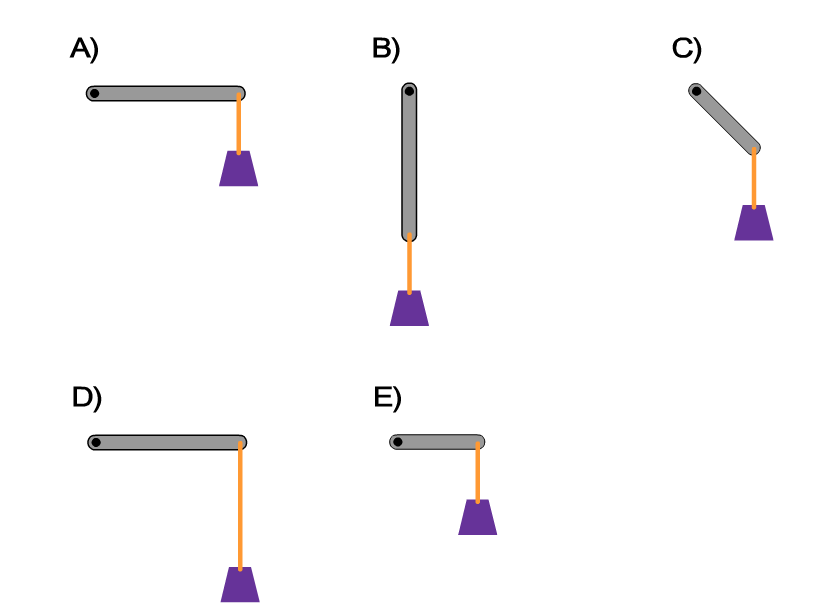In each of the five scenarios below, 1-kg mass is attached to the end of a massless stick by a massless string. At the momentum of release the sticks are motionless. Rank the torques acting on each stick due to the tension in the attached string. Take the axis of rotation to be the black dot on the left side of each stick.1. A>B>C>D>E
2. A=D>E>C>B
3. A=D=E>C>B
4. D=A=B>E>C
5. A=B=C=D=E